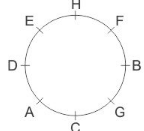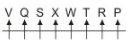Courses

# NABARD Manager Reasoning Mock Test 3

## 20 Questions MCQ Test NABARD Manager - Mock Tests & Previous Year Papers | NABARD Manager Reasoning Mock Test 3

Description
This mock test of NABARD Manager Reasoning Mock Test 3 for Banking Exams helps you for every Banking Exams entrance exam. This contains 20 Multiple Choice Questions for Banking Exams NABARD Manager Reasoning Mock Test 3 (mcq) to study with solutions a complete question bank. The solved questions answers in this NABARD Manager Reasoning Mock Test 3 quiz give you a good mix of easy questions and tough questions. Banking Exams students definitely take this NABARD Manager Reasoning Mock Test 3 exercise for a better result in the exam. You can find other NABARD Manager Reasoning Mock Test 3 extra questions, long questions & short questions for Banking Exams on EduRev as well by searching above.
QUESTION: 1

Solution:
QUESTION: 2

### In a march past,seven persons are standing in a row.Q is standing left to R but right to P.O is standing right to N and left to P.Similarly,S is standing right to R and left to T. Find out who is standing in middle

Solution: Q is left to R and to the right of P i.e. P, Q, R
O is to the right of N and left of P i.e. N, O, P
S is to the right of R and left of T i.e. R, S, T
So the order is : N, O, P, Q, R, S, T
Q is in the middle.
QUESTION: 3

### People tend to estimate the likelihood of an event's occurrence according to its salience; that is, according to how strongly and how often it comes to their attention. By placement and headlines, newspapers emphasize stories about local crime over stories about crime elsewhere and about many other major events. It can be concluded on the basis of the statements above that, if they are true, which of the following is most probably also true?

Solution:
QUESTION: 4

Paternalism is the practice by some governments of imposing regulations meant to promote their citizens’ own good regardless of whether the citizens consent to those regulations. However, many members of free societies disapprove when individual freedom is curtailed for the sake of what the government deems to be the citizens’ own good. They generally believe that they, not the government, know what activities are in their best interest.
If the statements above are true, which one of the following must also be true?

Solution:
QUESTION: 5

With information readily available on the Internet, consumers now often enter the automobile retail environment with certain models and detailed specifications in mind. In response to this trend, CarStore has decided to move toward a less aggressive sales approach. Despite the fact that members of its sales personnel have an average of ten years of experience each, CarStore has implemented a mandatory training program for all sales personnel, because __________.

Solution:
QUESTION: 6

In the following questions, the symbols \$, #, %, are used for different meanings as illustrated below:
'\$' means 'neither greater nor equal to'.
'#' means 'neither greater nor smaller than'.
'%' means 'neither smaller nor equal to'.
Now in each of the following questions assuming the given statements to be true, find out which of the two conclusions I and II given below them is/are definitely true.

Statements :
S \$ P, P % Q, Q # R
Conclusions :
I. R \$ S
II. R % S

Solution:
QUESTION: 7

In the following questions, the symbols \$, #, %, are used for different meanings as illustrated below:
'\$' means 'neither greater nor equal to'.
'#' means 'neither greater nor smaller than'.
'%' means 'neither smaller nor equal to'.
Now in each of the following questions assuming the given statements to be true, find out which of the two conclusions I and II given below them is/are definitely true.

Statements :
A \$ B, B # D, D % C
Conclusions :
I. D \$ A
II. B % C

Solution:
QUESTION: 8

In the following questions, the symbols \$, #, %, are used for different meanings as illustrated below:
'\$' means 'neither greater nor equal to'.
'#' means 'neither greater nor smaller than'.
'%' means 'neither smaller nor equal to'.
Now in each of the following questions assuming the given statements to be true, find out which of the two conclusions I and II given below them is/are definitely true.

Statements :
M # K, K % P, P \$ R
Conclusions :
I. R \$ M
II. P % M

Solution:
QUESTION: 9

Eight friends A, B, C, D, E, F, G and H are sitting around a circle facing the centre. H is to the immediate left of E who is third to the right of B. C is second to the right of D and is not a neighbour of B. F is second to the right of G and is not a neighbour of C.

Q. Who is second to the right of E?

Solution:QUESTION: 10

Eight friends A, B, C, D, E, F, G and H are sitting around a circle facing the centre. H is to the immediate left of E who is third to the right of B. C is second to the right of D and is not a neighbour of B. F is second to the right of G and is not a neighbour of C.

Q. Who is third to the left of A?

Solution:QUESTION: 11

Eight friends A, B, C, D, E, F, G and H are sitting around a circle facing the centre. H is to the immediate left of E who is third to the right of B. C is second to the right of D and is not a neighbour of B. F is second to the right of G and is not a neighbour of C.

Q. Which of the following pairs has the first person sitting to the immediate right of the second person?

Solution:QUESTION: 12

Each of the questions given below consists of a question and two statements numbered I and II given below it. You have to decide whether the data provided in the statements are sufficient to answer the questions.
On which day of week Mohan did reach Delhi?
Statements :
I. Mohan left Mumbai by train on Tuesday.
II. Mohan stayed for one day in Jaipur and completed his journey to Delhi in two days.

Solution:
QUESTION: 13

Each of the questions given below consists of a question and two statements numbered I and II given below it. You have to decide whether the data provided in the statements are sufficient to answer the questions.
D is in which direction of P?
Statements:
I. S is to south of P which is to the west of D.
II. P and R are in straight line and R is to the south of D.

Solution:
QUESTION: 14

Study the following information to answer the given questions:
P, Q, R, S, T, V, W and X are seated in a straight line facing North but not necessarily in the same order. P sits fourth to the right of X. Either P or X sit at the extreme ends of the line. Q and V are immediate neighbours of each other but none of them is an immediate neighbour of X and P. Only two people sit between S and T. The seating arrangement is such that W never sits at any of the positions towards the left of Q. W is not an immediate neighbour of P.

Q. What is the position of R with respect to X?

Solution:QUESTION: 15

A word arrangement machine, when given an input line of words, rearranges them following a particular rule in each step. The following is the illustration of the input and the steps of arrangement.
Input: man's mood varies with time and environment
Step I: varies with man's mood environment and time
Step II: and time environment mood man's varies with
Step III: environment time and varies with mood man's
Step IV: and varies environment time man's mood with
And so on for subsequent steps. You have to find out the logic and answer the questions given below:

Q. If Step V reads "bees are sucking juice from colourful flowers", what would Step III read?

Solution:
QUESTION: 16

A word arrangement machine, when given an input line of words, rearranges them following a particular rule in each step. The following is the illustration of the input and the steps of arrangement.
Input: man's mood varies with time and environment
Step I: varies with man's mood environment and time
Step II: and time environment mood man's varies with
Step III: environment time and varies with mood man's
Step IV: and varies environment time man's mood with
And so on for subsequent steps. You have to find out the logic and answer the questions given below:

Q. If Step III reads "old streets of Calcutta attract me lots", what would be the arrangement for Step VII?

Solution:
QUESTION: 17

A word arrangement machine, when given an input line of words, rearranges them following a particular rule in each step. The following is the illustration of the input and the steps of arrangement.
Input: man's mood varies with time and environment
Step I: varies with man's mood environment and time
Step II: and time environment mood man's varies with
Step III: environment time and varies with mood man's
Step IV: and varies environment time man's mood with
And so on for subsequent steps. You have to find out the logic and answer the questions given below:

Q. If Step IV reads "everyone were aware about their intimate friendship", what will be the middle three words of Step II?

Solution:
QUESTION: 18

Below are the statements followed by two conclusions numbered I and II. You have to consider the statements and the following conclusions and decide which of the conclusion(s) follows the statement(s).

Statements:
Some leaves are roots.
Some roots are stems.
All flowers are stems.
Conclusions:
I. No flower is a leaf.
II. Some leaves are stems.

Solution:

Some leaves are roots + Some roots are stems = I + I = No conclusion. Hence II and consequently do not follow.

QUESTION: 19

Below are the statements followed by two conclusions numbered I and II. You have to consider the statements and the following conclusions and decide which of the conclusion(s) follows the statement(s).

Statements:
All numbers are digits.
All alphabets are numbers.
All words are alphabets.
Conclusions:
I. All words are digits.
II. Some numbers are not words.

Solution:

All words are alphabets + All alphabets are numbers = A + A = A = All words are numbers → conversion → Some numbers are words (I). Hence II not follow. All words are numbers + All numbers are digits = A + A = A = All words are digits. Hence I follows.

QUESTION: 20

Below are the statements followed by two conclusions numbered I and II. You have to consider the statements and the following conclusions and decide which of the conclusion(s) follows the statement(s).

Statements:
All plastic are wood.
No wood is a metal.
Some metals are gases.
Conclusions:
I. No plastic is a metal.
II. Some gases are plastic.

Solution:

All plastics are wood + No wood is a metal = A+ E = E No plastic is a metal. Hence I follows. No plastic is a metal + Some metals are gases = E + I O* = Some gases are not plastic. Hence II does not follow.